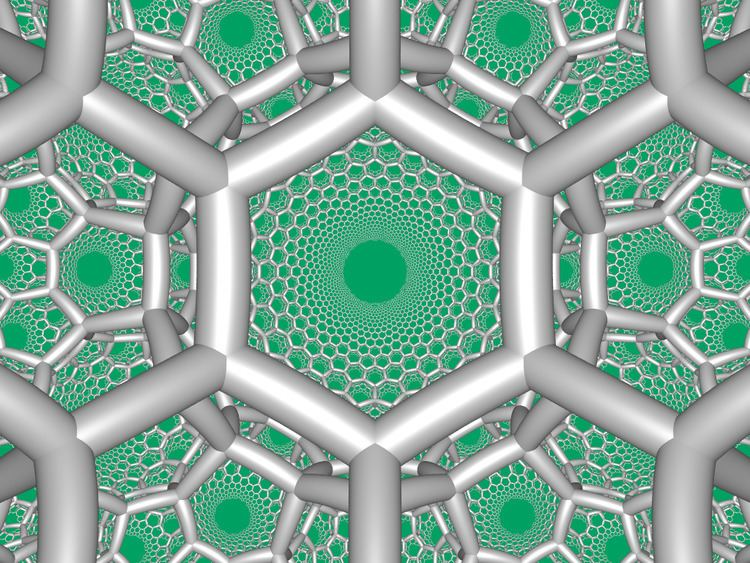Hexagonal tiling honeycomb

Updated onIn the field of hyperbolic geometry, the hexagonal tiling honeycomb arises one of 11 regular paracompact honeycombs in 3-dimensional hyperbolic space. It is called paracompact because it has infinite cells. Each cell consists of a hexagonal tiling whose vertices lie on a horosphere: a flat plane in hyperbolic space that approaches a single ideal point at infinity.

Contents

The Schläfli symbol of the hexagonal tiling honeycomb is {6,3,3}. Since that of the hexagonal tiling of the plane is {6,3}, this honeycomb has three such hexagonal tilings meeting at each edge. Since the Schläfli symbol of the tetrahedron is {3,3}, the vertex figure of this honeycomb is an tetrahedron. Thus, six hexagonal tilings meet at each vertex of this honeycomb, and four edges meet at each vertex.

Viewed in perspective outside of a Poincaré disk model, this shows one hexagonal tiling cell within the honeycomb, and its mid-radius horosphere (the horosphere incident with edge midpoints). In this projection, the hexagons grow infinitely small towards the infinite boundary asymptoting towards a single ideal point. It can be seen as similar to the order-3 apeirogonal tiling, {∞,3} of H2, with horocycle circumscribing vertices of apeirogonal faces.

Symmetry constructions

It has a total of five reflectional constructions from five related Coxeter groups all with four mirrors and only the first being regular: [6,3,3], [3,6,3], [6,3,6], [6,3] and [3[3,3]] , having 1, 4, 6, 12 and 24 times larger fundamental domains respectively. In Coxeter notation subgroup markups, they are related as: [6,(3,3)*] (remove 3 mirrors, index 24 subgroup); [3,6,3*] or [3*,6,3] (remove 2 mirrors, index 6 subgroup); [1+,6,3,6,1+] (remove two orthogonal mirrors, index 4 subgroup); all of these are isomorphic to [3[3,3]]. The ringed Coxeter diagrams are , , , and , representing different types (colors) of hexagonal tilings in the Wythoff construction.

Related polytopes and honeycombs

It is one of 15 regular hyperbolic honeycombs in 3-space, 11 of which like this one are paracompact, with infinite cells or vertex figures.

It is one of 15 uniform paracompact honeycombs in the [6,3,3] Coxeter group, along with its dual, the order-6 tetrahedral honeycomb, {3,3,6}.

Polytopes and honeycombs with tetrahedral vertex figures

It is in a sequence with regular polychora: 5-cell {3,3,3}, tesseract {4,3,3}, 120-cell {5,3,3} of Euclidean 4-space, with tetrahedral vertex figures.

Polytopes and honeycombs with hexagonal tiling cells

It is a part of sequence of regular honeycombs of the form {6,3,p}, with hexagonal tiling cells:

Rectified hexagonal tiling honeycomb

The rectified hexagonal tiling honeycomb, t1{6,3,3}, has tetrahedral and trihexagonal tiling facets, with a triangular prism vertex figure. The half-symmetry construction alternate two types of tetrahedra.

Truncated hexagonal tiling honeycomb

The truncated hexagonal tiling honeycomb, t0,1{6,3,3}, has tetrahedral and truncated hexagonal tiling facets, with a tetrahedral vertex figure.

It is similar to the 2D hyperbolic truncated order-3 apeirogonal tiling, t{∞,3} with apeirogonal and triangle faces:

Bitruncated hexagonal tiling honeycomb

The bitruncated hexagonal tiling honeycomb or bitruncated order-6 tetrahedral honeycomb, t1,2{6,3,3}, has truncated tetrahedra and hexagonal tiling cells, with a tetrahedral vertex figure.

Cantellated hexagonal tiling honeycomb

The cantellated hexagonal tiling honeycomb, t0,2{6,3,3}, has octahedral and rhombitrihexagonal tiling cells, with a triangular prism vertex figure.

Cantitruncated hexagonal tiling honeycomb

The cantitruncated hexagonal tiling honeycomb, t0,1,2{6,3,3}, has truncated tetrahedron and truncated trihexagonal tiling cells, with a tetrahedron vertex figure.

Runcinated hexagonal tiling honeycomb

The runcinated hexagonal tiling honeycomb, t0,3{6,3,3}, has tetrahedron, rhombitrihexagonal tiling hexagonal prism, triangular prism cells, with a octahedron vertex figure.

Runcitruncated hexagonal tiling honeycomb

The runcitruncated hexagonal tiling honeycomb, t0,1,3{6,3,3}, has cuboctahedron, Triangular prism, Dodecagonal prism, and truncated hexagonal tiling cells, with a quad-pyramid vertex figure.

Runcicantellated hexagonal tiling honeycomb

The runcicantellated hexagonal tiling honeycomb or runcitruncated order-6 tetrahedral honeycomb, t0,2,3{6,3,3}, has truncated tetrahedron, hexagonal prism, hexagonal prism, and rhombitrihexagonal tiling cells, with a quad-pyramid vertex figure.

Omnitruncated hexagonal tiling honeycomb

The omnitruncated hexagonal tiling honeycomb or omnitruncated order-6 tetrahedral honeycomb, t0,1,2,3{6,3,3}, has truncated octahedron, hexagonal prism, dodecagonal prism, and truncated trihexagonal tiling cells, with a quad-pyramid vertex figure.

References

Similar Topics
Inga Ålenius
David Popescu (politician)
Dani Stevenson
Topics